# Python Iterators: The Complete Guide

Python lists, tuples, dictionaries, and sets are all examples of inbuilt iterators. Again, Iterators are the objects that can be iterated upon.

## Python Iterators

Python iterators are objects that contain a countable number of values. An iterator is an object that can be iterated upon, meaning that you can traverse through all the values inside an iterator. Iterator is any Python type that can be used with a ‘for in loop.’

You can build your iterator using __iter__ and __next__ methods. In Python, an iterator is an object which implements the iterator protocol, which consists of __iter__ and __next__ methods.

Iterators are everywhere in the Python-like List, Tuple, Dictionary, Set, etc. They are elegantly implemented within the for loops, comprehensions, generators, etc. but hidden in plain sight.

An iterator in Python Programming Language merely is an object that can be iterated upon. An object which will return the data, one element at a time.

## Difference between Iterator and Iterable

Lists, tuples, dictionaries, and sets are all iterable objects. They are iterable containers that you can get an iterator from. All these objects have an iter() method used to get an iterator. Let’s take the example of an iterator.

```# app.py

listK = ['PS4', 'XBOX360', 'PSP']
itreables = iter(listK)

print(next(itreables))
print(next(itreables))
print(next(itreables))```

See the output.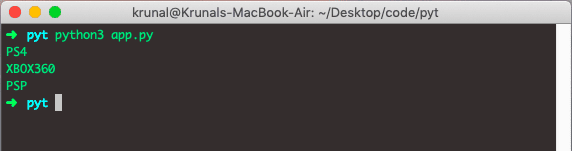Here, I have defined one list and then passed the list to the iter() function. Which returns the iterator we call the next() method, which will return the one item.

The __iter__ method is called on the initialization of an iterator. This should return an object with a next or __next__ (in Python 3) method.

The __next__  method should return the next value for the iterable. When an iterator is used with a ‘for in’ loop, the for loop implicitly calls next() on the iterator object. Therefore, this method should raise a StopIteration to signal the end of the iteration.

Let’s take a string as an example because strings are also iterables.

```# app.py

stringK = 'KRUNAL'
itreables = iter(stringK)
print(next(itreables))
print(next(itreables))
print(next(itreables))
print(next(itreables))
print(next(itreables))
print(next(itreables))```

See the output.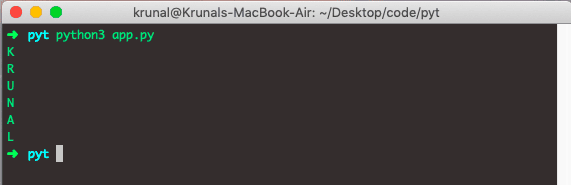Let’s see the tuple as an iterator example.

```# app.py

tupleK = ('Zuck', 'Jeff', 'Bill')
itreables = iter(tupleK)
print(next(itreables))
print(next(itreables))
print(next(itreables))```

See the output.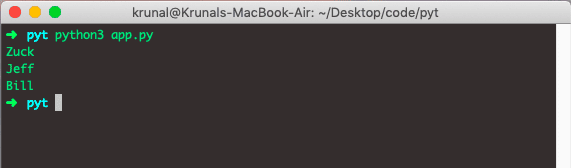## Create an Iterator in Python

If we want to create an object/class as an iterator, you have to implement the methods __iter()__ and __next()__ to your object.

The __iter()__ method acts similarly. You can do operations (initializing, etc.) but must always return the iterator object itself. The __next()__ method also allows you to do operations and must return the next item in the sequence. See the following example.

```# app.py

class App:
def __iter__(self):
self.number = 1
return self

def __next__(self):
data = self.number
self.number += 1
return data

IClass = App()
myiter = iter(IClass)

print(next(myiter))
print(next(myiter))
print(next(myiter))
print(next(myiter))
print(next(myiter))
print(next(myiter))```

In the above example, I have defined one class App and then defined two methods __iter()__ and __next()__. The __iter()__ function returns the number, and then __next()__ function increments the number by 1.

See the output.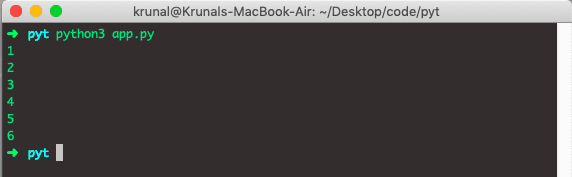## Iterating Through an Iterator in Python

We use the next() method to iterate through an iterator’s elements manually. When we reach the end, and there is no more data to be returned, it will raise StopIteration.

See the following example.

```# app.py

my_list = [21, 19, 29, 46]
my_iter = iter(my_list)
print(next(my_iter))
print(next(my_iter))
print(my_iter.__next__())
print(my_iter.__next__())
next(my_iter)
```

See the output.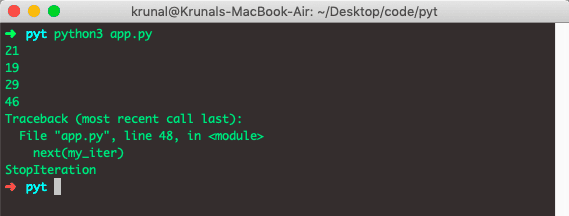When all the items are iterated, and you still want to iterate the next item which is not there in the list, then it will throw an exception and tell us that StopIteration.

That’s it for this tutorial.

### 1 thought on “Python Iterators: The Complete Guide”

1.Thanks for the post